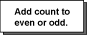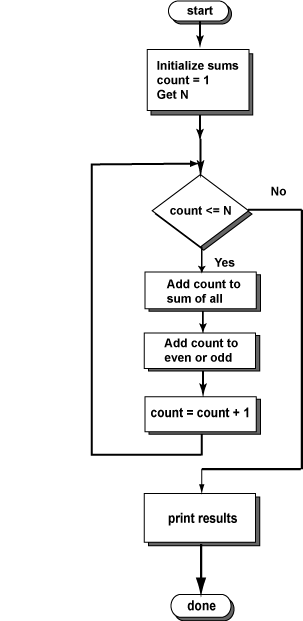Probably not. Even with a calculator this would be tedious.

There is a formula for the sum of integers from 1 to N and similar formulas for the sum of even or of odd integers from 1 to N, but pretend you don't know that. Let us write a program that calculates these three sums by directly adding up the integers:

• The sum of all integers 1 to N
• The sum of odd integers 1 to N
• The sum of even integers 1 to N

The user enters N, and the program counts up from one to N adding each integer to the appropriate sums. The flowchart shows how the program works.

The flowchart shows a loop that increments `count` from 1 to N. In the loop body, the current value of `count` is always added to the sum of all integers.

But more work needs to be done. `count` should be added to the sum of odds only if it is odd and added to the sum of evens only if it is even.

This box:needs more detail.### QUESTION 2:

How can you decide if `count` should be added to the sum of even or to the sum of odd integers?